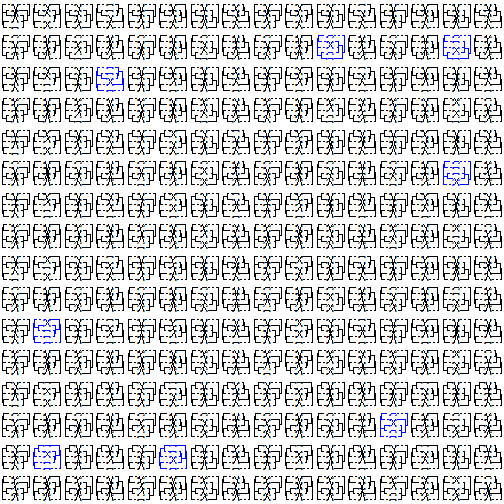## Saturday, October 1, 2016

### Evaluating all possible co-phylogenetic plots for a pair of trees

I just pushed an update to the co-phylogenetic phytools method `cophylo` to permit it to compute & return all possible rotated objects of class `"cophylo"` for a pair of trees and table of associations.

This is functionally equivalent to using `allRotations` to rotate both trees in all possible ways, and then `cophylo` to compute an object of class `"cophylo"` (without rotation) on each pair of trees.

This will generally result in a large number of `"cophylo"` objects for even a modest number of taxa in the two input trees, as follows:

``````X
``````
``````##    N1=N2=   N(cophylo)
## 1       2            4
## 2       3           16
## 3       4           64
## 4       5          256
## 5       6         1024
## 6       7         4096
## 7       8        16384
## 8       9        65536
## 9      10       262144
## 10     11      1048576
## 11     12      4194304
## 12     13     16777216
## 13     14     67108864
## 14     15    268435456
## 15     16   1073741824
## 16     17   4294967296
## 17     18  17179869184
## 18     19  68719476736
## 19     20 274877906944
``````

Let's try the update:

``````library(phytools)
t1<-rtree(n=5,tip.label=LETTERS[1:5])
t2<-rtree(n=5,tip.label=letters[1:5])
assoc<-cbind(LETTERS[1:5],letters[1:5])
obj<-cophylo(t1,t2,methods="all",assoc=assoc)
``````
``````## Rotating nodes to optimize matching...
## Done.
``````
``````match.score<-sapply(obj,function(x) sum(attr(x\$trees[],"minRotate"),
attr(x\$trees[],"minRotate")))
best<-which(match.score==min(match.score))

par(mfrow=c(16,16))
par(mar=rep(0,4))

for(i in 1:length(obj)){
if(i%in%best) par(fg="blue") else par(fg="black")
plot(obj[[i]],
pts=FALSE,ylim=c(-0.1,1.1))
}
``````Here I have plotted the best matching rotations in blue.

That's it.